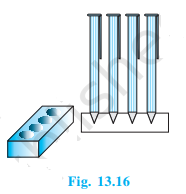# A pen stand made of wood is in the shape of a cuboid with four conical depressions to hold pens. The dimensions of the cuboid are 15 cm by 10 cm by 3.5 cm. The radius of each of the depressions is 0.5 cm and the depth is 1.4 cm. Find the volume of wood in the entire stand (see Fig.) .Given

Length of cuboid (l) = 15 cm

Breadth of cuboid (b) = 10 cm

Height of cuboid (h) = 3.5 cm

Radius of conical part (r) = 0.5 cm

Height of conical part (H) = 1.4 cm

Find out

We have to find out the volume of wood in the entire stand

Solution

Total Volume wood in the entire stand = Volume of Cuboid – Volume of four conical part

= (l × b × h) – 4 × (1/3πr2H)

On considering π=22/7 we get

= (15 × 10 × 3.5) – (4 × (1/3)(22/7) × 0.5 × 0.5 × 1.4)

= 525 – (1.466)

= 523.5333 cm3

The volume of wood= 523.5333 cm3(1)(0)